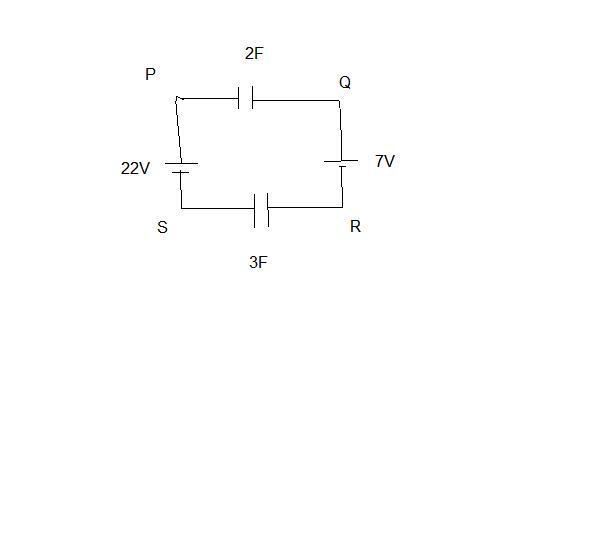# Potential difference across a capacitor problem

## Homework Statement

The problem is to find out the potential difference between P and Q in the following figure-The solution given takes the charges on two capacitors to be equal. Why is it so?

#### Attachments

gneill
Mentor
It's a series circuit, so any current that flows while the circuit heads to equilibrium must be the same for all components. Same current --> same amount of charge moved.

Some amount of charge say Q1 may flow from the 22V battery to the 7V battery to charge the 2F capacitor. Q2 amount may flow from 7V battery to 22V battery. How can we say that the charges are equal unless the two capacitances are equal?

gneill
Mentor
The current in a series circuit is everywhere the same. Any current that leaves the 22V battery's + terminal must also flow back into its - terminal. Same goes for the 7V battery, and all the other components in the series.

I got it now. Thanks!